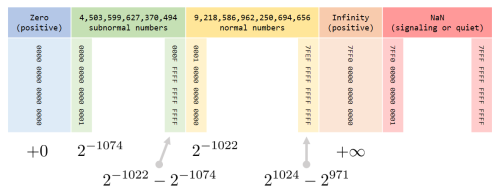# 浮点数的二进制分布

(((std::uint32_t&)a)&0x7FFFFFFF) < (((std::uint32_t&)b)&0x7FFFFFFF)# RANSAC 和一个有趣的 bug

size_t iteration_limit(double r, double P, size_t k) {
double n = log(1-P) / log(1-pow(r, k));
return (size_t)ceil(n);
}


size_t iteration_limit(double r, double P, size_t k) {
double n = log(1-P) / log(1-pow(r, k));
return (size_t)ceil(std::min(1.0e10, n));
}


1. Multiple View Geometry in Computer Vision, second edition. Hartley, R. I. and Zisserman, A., Cambridge University Press, 2014.